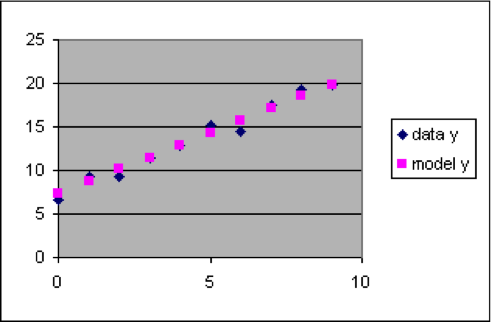## I1.02: Section 1

### Section 1: Graphing data and model together

In order to find a good model for a dataset, we need to be able to compare the actual data values with the predictions of the model. This can be done by applying the model formula to each of the x values in Column A to compute “model y” values that are placed in Column C next to the corresponding “data y” value in Column B. Then a scatter plot that is made with all three columns selected will show both the data and the model predictions, in different colors. For a good model, the two kinds of points will be close to each other, although the data points will usually also include some random noise.

### Example 1—Adding a model to a dataset and setting up a comparison graph

 Input Output x data y 0 6.6 1 9.3 2 9.2 3 11.5 4 12.9 5 15.2 6 14.4 7 17.5 8 19.3 9 19.8
The dataset to the left has a relationship between x and y that is approximated by the linear formula y = 1.4 x + 7.3. This formula can be used to compute model y values for each of the rows of the dataset, which we will put into column C next to the corresponding output data y value so that we can easily compare them.

1. Insert a new worksheet into a spreadsheet.
2. Copy the dataset so that the x and y values go into columns A and B, with the numbers starting in row 3.         (That is, cell A3 will be 0 and B3 will be 7.41)
3. Put the labels “Model” into cell C1 and “model y” into cell C2.
4. Put the formula “=1.4*A3+7.3” into cell C3. (The result for C3 should be 7.3)
5. Spread the formula in C3 down column C to C12, next to all the data values in column B. (The results should be 8.7 for C4, 10.1 for C5, 11.5 for C6, etc.)
6. Select the rectangle from A2 to C12, and make a scatter plot.

When you have followed the steps listed above, you should have results that look about like this:

 A B C D E F G H I 1 Input Output Prediction 2 x data y model y 3 0 6.6 7.3 4 1 9.3 8.7 5 2 9.2 10.1 6 3 11.5 11.5 7 4 12.9 12.9 8 5 15.2 14.3 9 6 14.4 15.7 10 7 17.5 17.1 11 8 19.3 18.5 12 9 19.8 19.9 13The graph above shows that y = 1.4 x + 7.3 is a good model for this dataset, since the data points are close to the model points over the entire range of data, and the differences are randomly above and below the model.

But how did we know that the right model was y = 1.4 x + 7.3?

Good question. The example above shows how to recognize when a formula makes a good model, but does not show how to find a good model formula. What we really want is a method that will permit us to take any dataset that seems linear and quickly find what particular values for the slope and intercept parameters will make a linear formula that is a good model for that dataset (or a similar process for an appropriate nonlinear formula if the data pattern is not close to a straight line). The next section introduces a tool that provides an easy way to find the right parameter settings in a model formula.